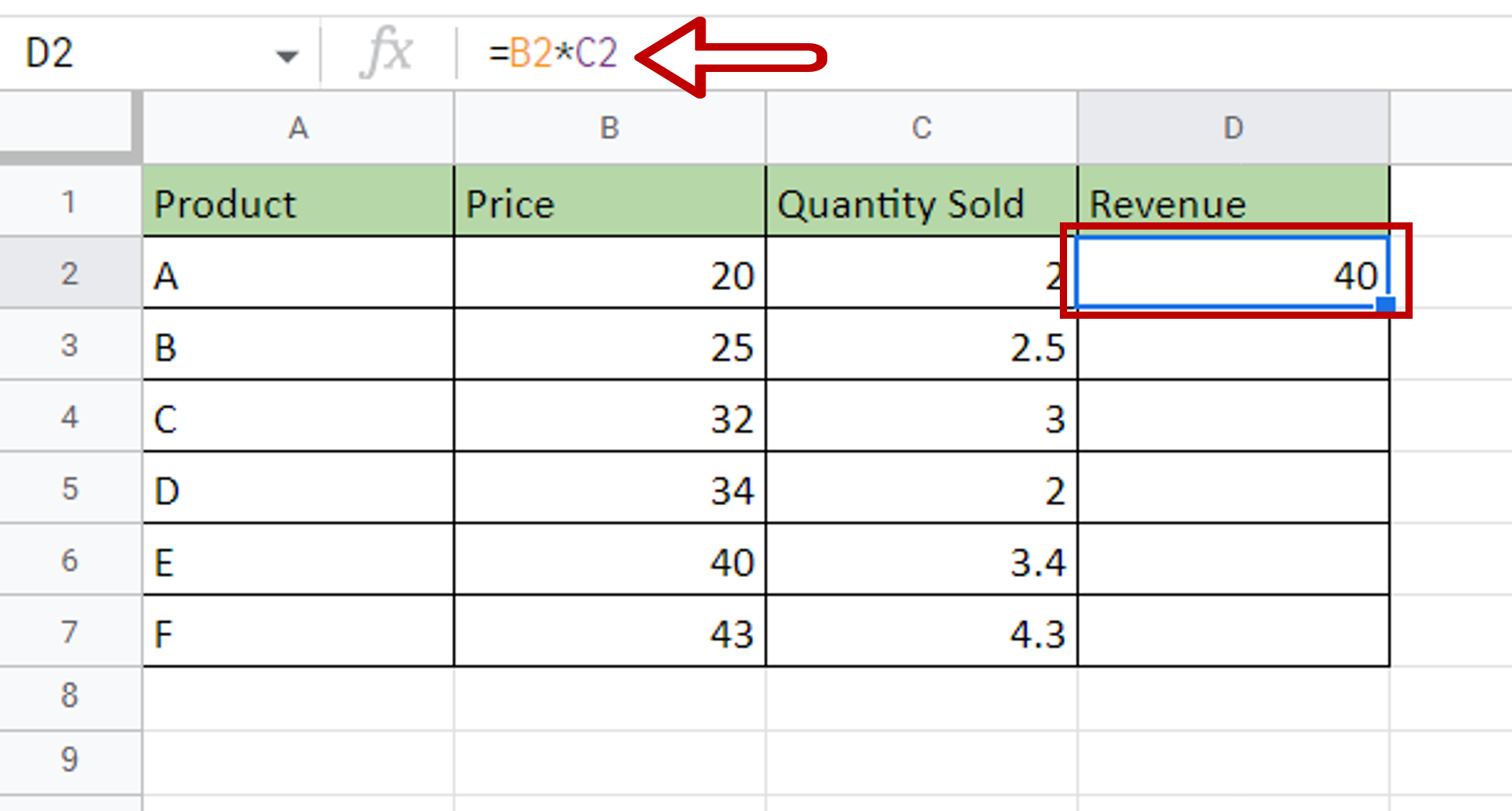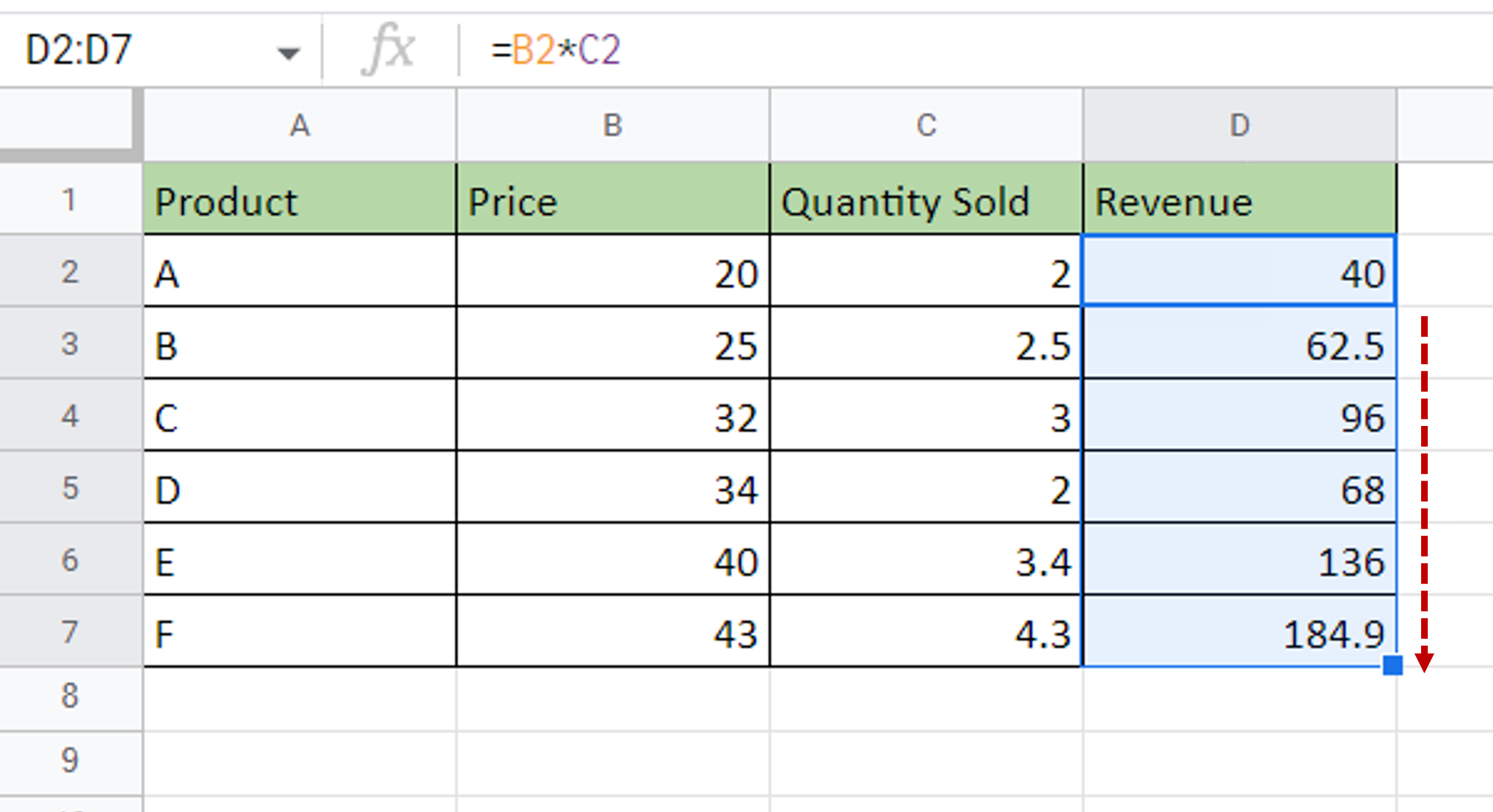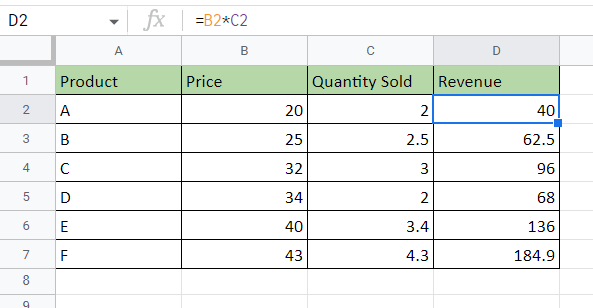# How to multiply columns in Google Sheets

You can watch a video tutorial here.Google Sheets is widely used for calculations due to the several arithmetic operators and functions that it has. An advantage of creating formulas in Google Sheets is that they can be created using cell references. A formula created in a cell, using cell references, can be copied to the rest of the column and the cell references will change according to the direction in which the copying is done. When copying a formula down a column, the row number will increment accordingly. To multiply columns in Google Sheets, the formula is created in the first cell and then copied to the rest of the column.

### Step 1 – Enter the formula– Select the first cell of the column where the result is to be displayed
– Type the formula using cell references:
=Price * Quantity sold

### Step 2 – Copy the formula– Using the fill handle from the first cell, drag the formula to the remaining cells
OR
a) Select the cell with the formula and press Ctrl+C or choose Copy from the context menu (right-click)
b) Select the rest of the cells in the column and press Ctrl+V or choose Paste from the context menu (right-click)

### Step 3 – Check the result– The ‘Price’ and ‘Quantity Sold’ columns are multiplied and the result is displayed in the ‘Revenue’ column